### Become an OU studentStart this free course now. Just create an account and sign in. Enrol and complete the course for a free statement of participation or digital badge if available.

# 3.2 Quartiles and the interquartile range

Finding the quartiles of a batch is very similar to finding the median.

In Subsection 1.2, we represented a batch as a V-shaped formation, with the median at the ‘hinge’ where the two arms of the V meet. The median splits the batch into two equal parts. Similarly, we can put another hinge in each side of the V and get four roughly equal parts, shaped like this: . For a batch of size 15, it looks like Figure 12.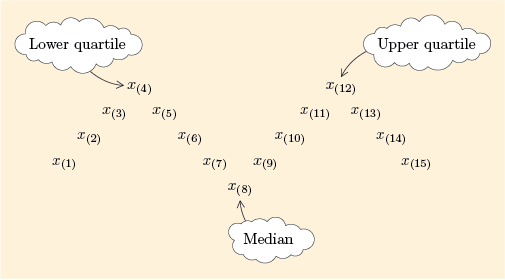Figure 12 Median and quartiles

The points at the side hinges, in this case and , are the quartiles. There are two quartiles which, as with the extremes, we call the lower quartile and the upper quartile. The lower quartile separates off the bottom quarter, or lowest 25%. The upper quartile separates off the top quarter, or highest 25%. They are denoted and respectively. (Sometimes they are referred to as the first quartile and the third quartile.)

You might be wondering, if these are and , what happened to ? Well, have a think about that for a moment.

separates the bottom quarter of the data (from the top three quarters), and separates the bottom three quarters (from the top quarter). So it would make sense to say that separates the bottom two quarters (from the top two quarters). But two quarters make a half, so would denote the median, and since there is already a separate word for that, it’s not usual to call it the second quartile.

Usually we cannot divide the batch exactly into quarters. Indeed, this is illustrated in Figure 12 where the two central parts of the are larger than the outer ones. As with calculating the median for an even-sized batch, some rule is needed to tell us how many places we need to count along from the smallest value to find the quartiles. However, there are several alternatives that we could adopt and the particular rule described below is somewhat arbitrary. Different authors and different software may use slightly different rules. If your calculator can find quartiles, note that it may use a different rule.

As you might have expected, the rule involves dividing by 4, where is the batch size (as opposed to dividing by 2 to find the median). However, the rule is slightly more complicated for the quartiles and it depends on whether is exactly divisible by 4.

## The quartiles

The lower quartile is at position in the ordered batch.

The upper quartile is at position in the ordered batch.

If is exactly divisible by 4, these positions correspond to a single value in the batch.

If is not exactly divisible by 4, then the positions are to be interpreted as follows.

• A position which is a whole number followed by means ‘halfway between the two positions either side’ (as was the case for finding the median).

• A position which is a whole number followed by means ‘one quarter of the way from the position below to the position above’. So for instance if a position is , the quartile is the number one quarter of the way from to .

• A position which is a whole number followed by means ‘three quarters of the way from the position below to the position above’. So for instance if a position is , the quartile is the number three quarters of the way from to .

Before we actually use these rules to find quartiles, let us look at some more examples of -shaped diagrams for different batch sizes . The case where is exactly divisible by 4, so that is a whole number, was shown in Figure 12. The following three figures show the three other possible scenarios, where is not exactly divisible by 4.

For , and . So is halfway between and , and is halfway between and .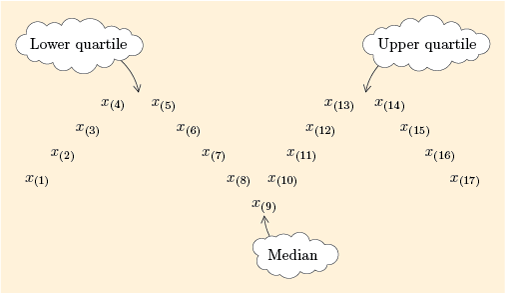Figure 13 Quartiles for sample size

For , and . So is three quarters of the way from to , and is one quarter of the way from to .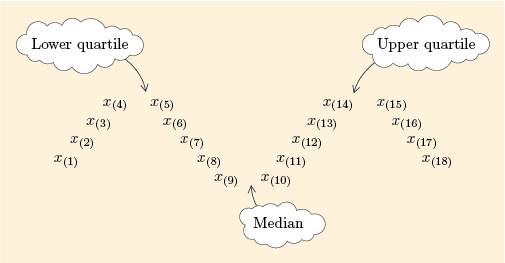Figure 14 Quartiles for sample size

For , and . So is one quarter of the way from to , and is three quarters of the way from to .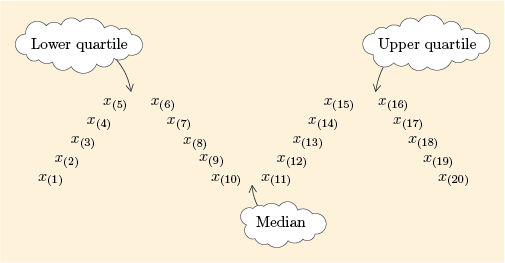Figure 15 Quartiles for sample size

## Example 14 Quartiles for the prices of small televisions

Figure 15 showed you where the quartiles are for a batch of size 20. Let us now use the stemplot of the 20 television prices in Figure 16, which you first met in Figure 5 (Subsection 1.2), to find the lower and upper quartiles, and , of this batch.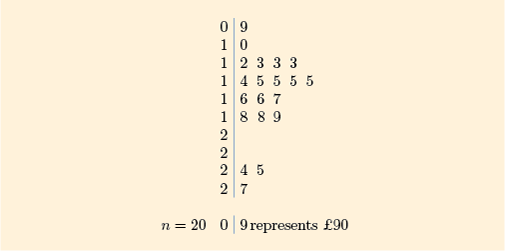Figure 16 Prices of flat-screen televisions with a screen size of 24 inches or less

To calculate the lower quartile you need to find the number that is one quarter of the way from to . These values are both 130, so is 130. To calculate the upper quartile you need to find the number three quarters of the way from to . These values are both 180, so is 180.

That example was easier than it might have been, because for each quartile the two numbers we had to consider turned out to be equal!

## Example 15 Quartiles for the camera prices

Table 2 (Subsection 1.2) gave ten prices for a particular model of digital camera (in pounds). In order, the prices are as follows.

To find the lower and upper quartiles, and , of this batch, first find and .

The lower quartile is the number three quarters of the way from to . These values are 60 and 65. The difference between them is , and three quarters of that difference is . Therefore is 3.75 larger than 60, so it is 63.75. As with the median, in this course we will generally round the quartiles to the accuracy of the original data, so in this case we round to the nearest whole number, 64. In symbols, .

The upper quartile is the number one quarter of the way from to . These values are 81 and 85. The difference between them is , and one quarter of that difference is . Therefore is 1 larger than 81, so it is 82. (No rounding necessary this time.) In symbols, .

Example 15 is the subject of the following screencast. [Note that references to ‘the unit’ should be interpreted as ‘this course’. The original wording refers to the Open University course from which this material is adapted.]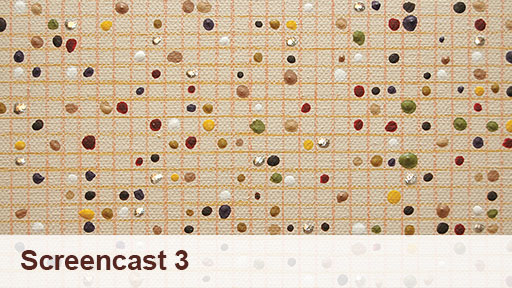Screencast 3 Calculating quartiles
Interactive feature not available in single page view (see it in standard view).

## Activity 10 Finding more quartiles

(a) Find the lower and upper quartiles of the batch of 15 coffee prices in Figure 17. (This batch of coffee prices was first introduced in Table 1 of Subsection 1.1.)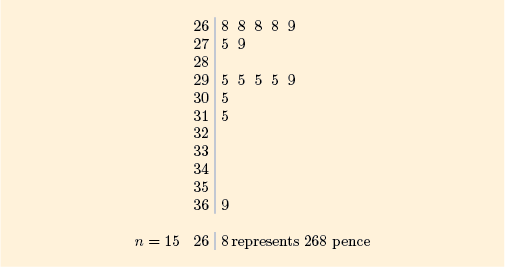Figure 17 Stemplot of 15 coffee prices

### Discussion

Here, because , an appropriate picture of the data would be Figure 12 (Subsection 3.2). To find the lower and upper quartiles, and , of this batch, first find and . Therefore and .

(b) Find the lower and upper quartiles of the batch of 14 gas prices in Figure 18. (This batch of gas prices was first introduced in Table 3 of Subsection 1.2.)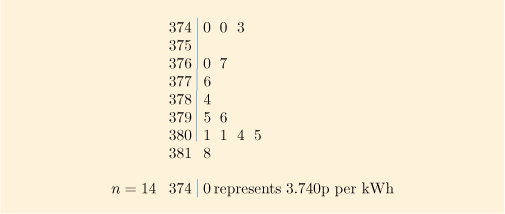Figure 18 Stemplot of 14 gas prices

### Discussion

For this batch, so and .

and

So the lower quartile is 3.756 p per kWh and the upper quartile is 3.802p per kWh.